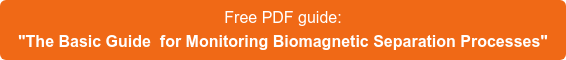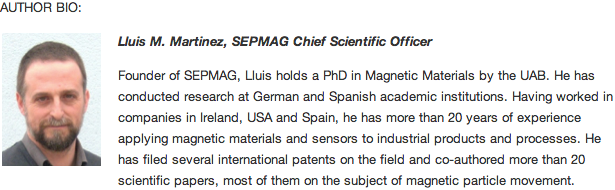Blog

The purpose obtain a record of different processes to be able to compare the results. Using homogenous magnetic force, changes can easily be related to any modifications made to the suspension. Changes to the magnetic bead specifications (diameter, magnetic moment), concentration, and variations in buffer viscosity suggest different separation process dynamics. As discussed in previous articles, the traditional method for monitoring the biomagnetic separation process is to measure the suspension opacity. However, in order to have a systematic, objective comparison method, it is desirable to parameterize the resultant curve.

When SEPMAG started monitoring biomagnetic separation processes a decade ago, we assumed that the behavior would be an exponential decay. But our early experiments revealed a poor fit between the experimental data with this expression. This discrepancy forced us to do more research into the mechanisms underlying the process. Based on the premise of the constant magnetic force, researchers of UAB and ICMAB-CSIC helped us to understand the cooperative nature of the biomagnetic separation process and why the separation does not decay exponentially [Andreu et al, Journal of Nanomaterials Article ID: 678581 (2012)].

Further experimental work demonstrated that the typical biomagnetic separation curve can be successfully fitted by a sigmoidal function. The behavior can then be described by just four parameters: opacity at the starting time (when t=0), +final opacity (at t=infinite), the slope (defined by the dimensionless exponent ‘p’) and the half-separation time (t50, expressed in seconds).

A typical example is to determine whether the magnetic beads are aggregated –something that may happen if they have not been stocked in the right conditions. If the transparency is only checked at the specified separation time, good and bad batches will seem both OK, as the final state is exactly the same. However, the monitoring curves tell a different story. The separation of the second batch (lighter line) is faster than the original one. We can quantify the difference by taking the t50 value of the sigmoidal fitting: for beads acting as expected, the t50 is 32 seconds, but for the batch with aggregates the t50=22 s, which is 30% faster! Notice that irreversible aggregates act like larger diameter magnetic beads, meaning quicker separation. The differences between both batches after 100 s are very difficult to detect, because they are within the experimental error, but parameterizing the curve, t50 gives us a simple way to compare both processes and identify irreversible bead aggregation in the faster separation.Sigmoidal fitting also allows us normalize the measurements, making it easy to compare different magnetic beads concentrations. Using the experimental measurements, the starting opacity would be very different. Lower concentration means lower opacity. Using the opacity at t=infinite as zero and the opacity at t=0 as 100%, the comparison is straightforward. When the biomagnetic separation process is due to cooperative behavior (each bead interacts magnetically with its neighbors), lower concentration means weaker interactions and a slower process. In the example we can see how when the same magnetic beads are diluted from 1% to 0.1%, t50 increases from 7 s to 24 s. The slope of the sigmoidal also changes, becoming smoother when diluted. It changes from 2.7 to 2.0 (the graph shows different assays for each concentration).We can conclude that the curves obtained by optical monitoring of the biomagnetic separation at a constant magnetic force are a powerful tool for identifying changes in the process. But if the curves are fitted to a model, we can quantify the variations and use this information to correlate them with the changes in the suspension (beads or buffer).

Related articles: